## Percent

A fraction whose denominator is 100 is called a percent. The word percent means hundredths (per hundred).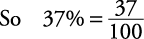#### Changing decimals to percents

To change decimals to percents,

1. Move the decimal point two places to the right.

2. Insert a percent sign.

##### Example 1

Change to percents.

1. 0.09 = 9%

2. 0.75 = 75%

3. 1.85 = 185%

4. 0.002 = 0.2%

5. 8.7 = 870%

#### Changing percents to decimals

To change percents to decimals,

1. Eliminate the percent sign.

2. Move the decimal point two places to the left (sometimes, adding zeros will be necessary).

##### Example 2

Change to decimals.

1.2.3.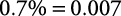4.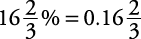#### Changing fractions to percents

There are two simple methods for changing fractions to percents.

1. Change to a decimal.

2. Change the decimal to a percent.

##### Example 3

Change to percents.

1.2.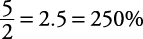3.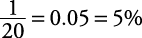1. Make a proportion with the fraction on the left side of the equal sign and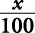on the right side of the equal sign.

2. Solve the proportion for x, then place a % sign to its right.

##### Example 4

Change to percents.

1.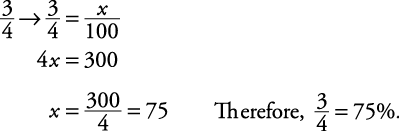2.3.#### Changing percents to fractions

There are two simple methods for changing percents to fractions.

1. Drop the percent sign.

2. Write over one hundred.

3. Reduce if necessary.

##### Example 5

Change to fractions.

1.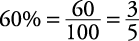2.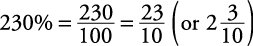1. Drop the percent sign.

2. Multiply by.

3. Reduce if necessary.

##### Example 6

Change to fractions.

1.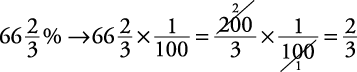2.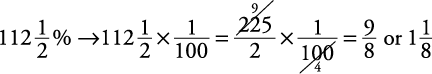Memorizing the following can eliminate computations: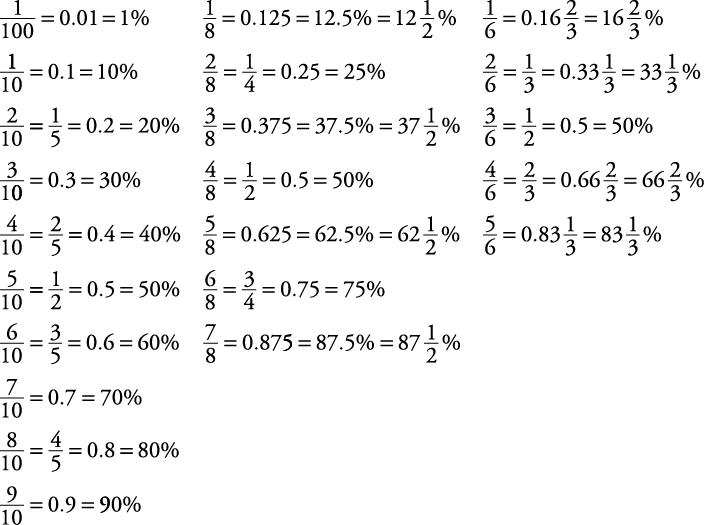#### Finding the percent of a number

To determine the percent of a number, change the percent to a fraction or decimal (whichever is easier for you) and multiply. Remember, the word of means multiply.

##### Example 7

Find the percents of these numbers.

1.2.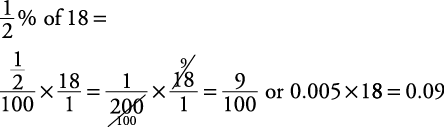#### Other applications of percent

Turn the question word‐for‐word into an equation. For what, substitute the letter x; for is, substitute an equal sign; for of, substitute a multiplication sign. Change percents to decimals or fractions, whichever you find easier. Then solve the equation.

##### Example 8

Turn each of the following into an equation and solve.

1. 18 is what percent of 90?2. 10 is 50 percent of what number?3. What is 15 percent of 60?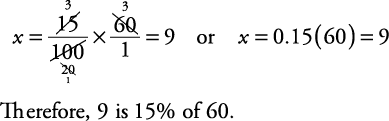#### Percent—proportion method

Another simple method commonly used to solve percent problems is the proportion or is/of method. First set up a blank proportion and then fill in the empty spaces by using the following steps.

This method, using proportions with is/of, works for the three basic types of percent questions.##### Example 9

30 is what percent of 50?

The “is” number is 30. The % number is unknown, so call it x.

The “of” number is 50. Now replace these values in the appropriate positions in the proportion and solve for x.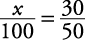In this particular instance, it can be observed thatso the answer is 60%. Solving mechanically on this problem would not be time effective.

##### Example 10

30 is 20% of what number?Therefore, 30 is 20% of 150.

##### Example 11

What number is 30% of 50?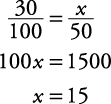Therefore, 15 is 30% of 50.